# Calulation of deflection and crack width according to is 456 2000

5 de Apr de 2020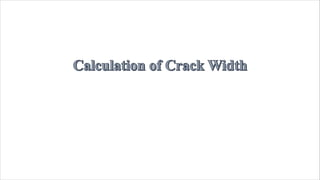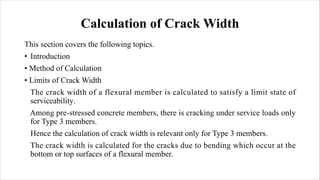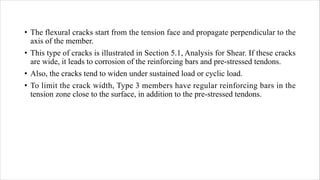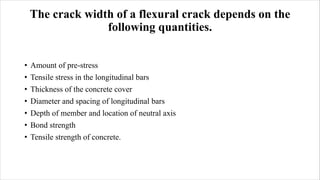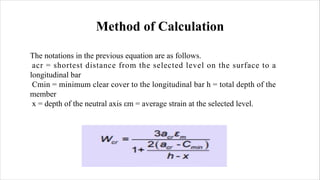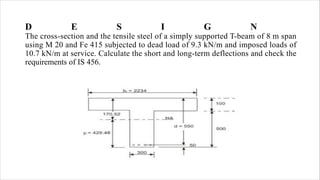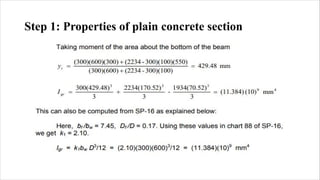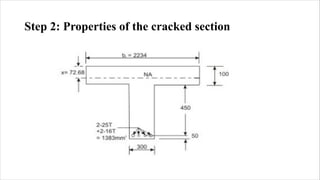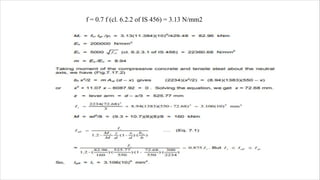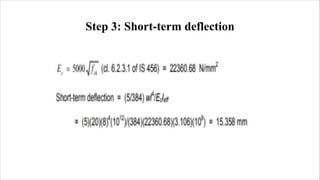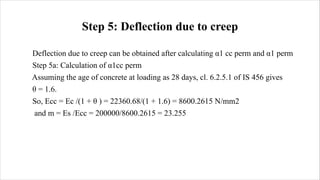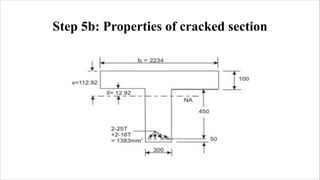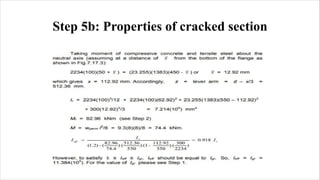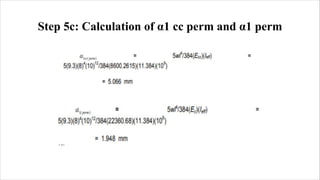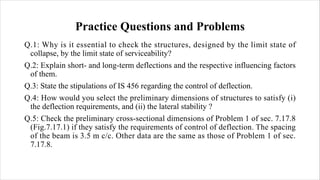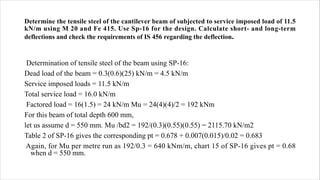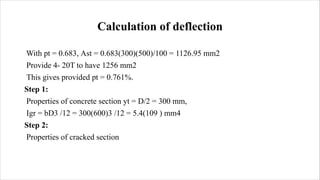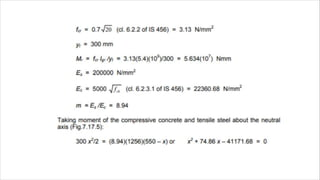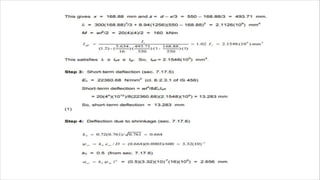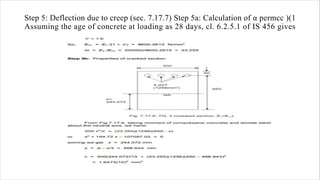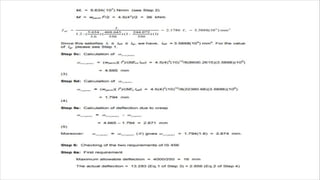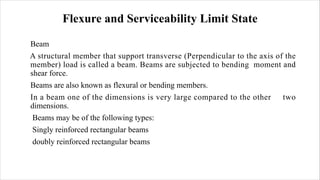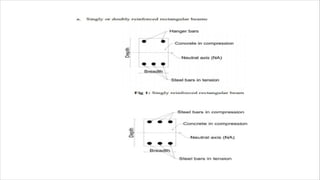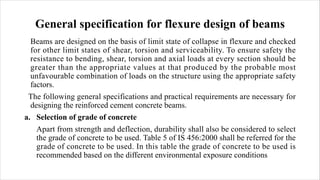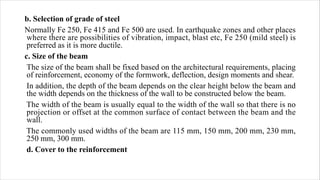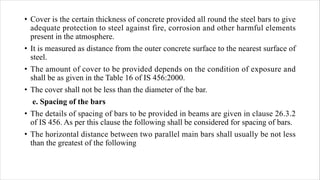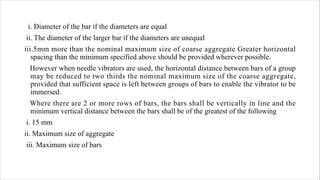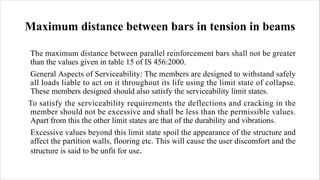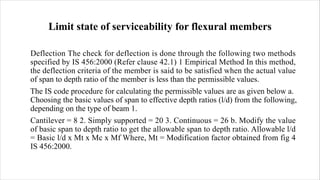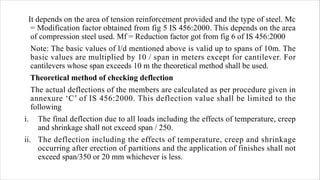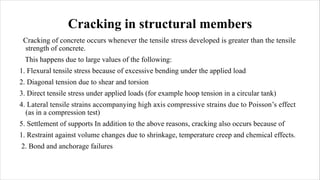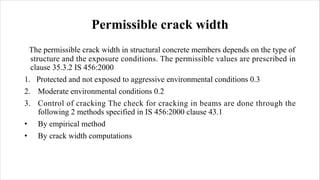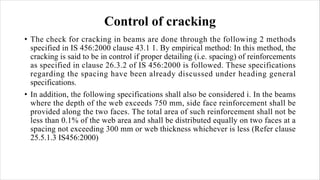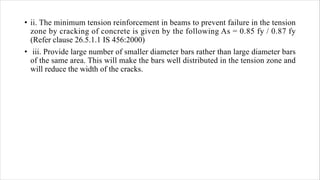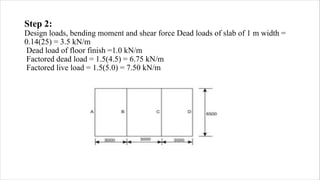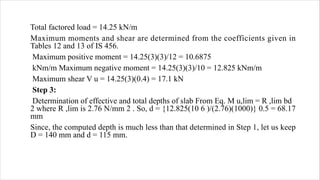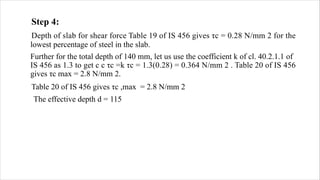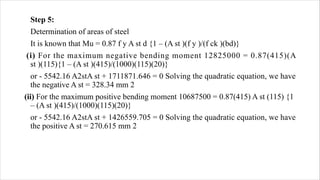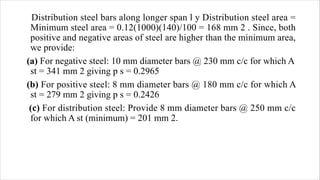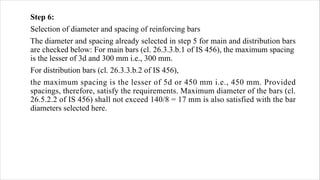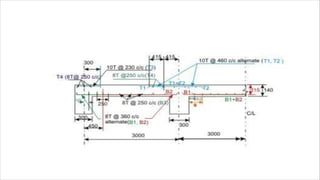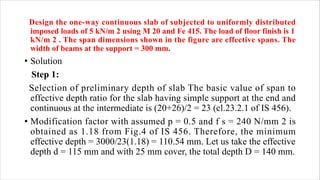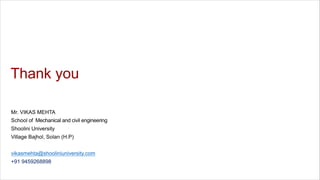1 de 51

### Calulation of deflection and crack width according to is 456 2000

• 2. Calculation of Crack Width This section covers the following topics. • Introduction • Method of Calculation • Limits of Crack Width The crack width of a flexural member is calculated to satisfy a limit state of serviceability. Among pre-stressed concrete members, there is cracking under service loads only for Type 3 members. Hence the calculation of crack width is relevant only for Type 3 members. The crack width is calculated for the cracks due to bending which occur at the bottom or top surfaces of a flexural member.
• 3. • The flexural cracks start from the tension face and propagate perpendicular to the axis of the member. • This type of cracks is illustrated in Section 5.1, Analysis for Shear. If these cracks are wide, it leads to corrosion of the reinforcing bars and pre-stressed tendons. • Also, the cracks tend to widen under sustained load or cyclic load. • To limit the crack width, Type 3 members have regular reinforcing bars in the tension zone close to the surface, in addition to the pre-stressed tendons.
• 4. The crack width of a flexural crack depends on the following quantities. • Amount of pre-stress • Tensile stress in the longitudinal bars • Thickness of the concrete cover • Diameter and spacing of longitudinal bars • Depth of member and location of neutral axis • Bond strength • Tensile strength of concrete.
• 5. Method of Calculation The notations in the previous equation are as follows. acr = shortest distance from the selected level on the surface to a longitudinal bar Cmin = minimum clear cover to the longitudinal bar h = total depth of the member x = depth of the neutral axis εm = average strain at the selected level.
• 6. D E S I G N The cross-section and the tensile steel of a simply supported T-beam of 8 m span using M 20 and Fe 415 subjected to dead load of 9.3 kN/m and imposed loads of 10.7 kN/m at service. Calculate the short and long-term deflections and check the requirements of IS 456.
• 7. Step 1: Properties of plain concrete section
• 8. Step 2: Properties of the cracked section
• 9. f = 0.7 f (cl. 6.2.2 of IS 456) = 3.13 N/mm2
• 10. Step 3: Short-term deflection
• 11. Step 4: Deflection due to shrinkage
• 12. Step 5: Deflection due to creep Deflection due to creep can be obtained after calculating α1 cc perm and α1 perm Step 5a: Calculation of α1cc perm Assuming the age of concrete at loading as 28 days, cl. 6.2.5.1 of IS 456 gives θ = 1.6. So, Ecc = Ec /(1 + θ ) = 22360.68/(1 + 1.6) = 8600.2615 N/mm2 and m = Es /Ecc = 200000/8600.2615 = 23.255
• 13. Step 5b: Properties of cracked section
• 14. Step 5b: Properties of cracked section
• 15. Step 5c: Calculation of α1 cc perm and α1 perm
• 16. Practice Questions and Problems Q.1: Why is it essential to check the structures, designed by the limit state of collapse, by the limit state of serviceability? Q.2: Explain short- and long-term deflections and the respective influencing factors of them. Q.3: State the stipulations of IS 456 regarding the control of deflection. Q.4: How would you select the preliminary dimensions of structures to satisfy (i) the deflection requirements, and (ii) the lateral stability ? Q.5: Check the preliminary cross-sectional dimensions of Problem 1 of sec. 7.17.8 (Fig.7.17.1) if they satisfy the requirements of control of deflection. The spacing of the beam is 3.5 m c/c. Other data are the same as those of Problem 1 of sec. 7.17.8.
• 17. Determine the tensile steel of the cantilever beam of subjected to service imposed load of 11.5 kN/m using M 20 and Fe 415. Use Sp-16 for the design. Calculate short- and long-term deflections and check the requirements of IS 456 regarding the deflection. Determination of tensile steel of the beam using SP-16: Dead load of the beam = 0.3(0.6)(25) kN/m = 4.5 kN/m Service imposed loads = 11.5 kN/m Total service load = 16.0 kN/m Factored load = 16(1.5) = 24 kN/m Mu = 24(4)(4)/2 = 192 kNm For this beam of total depth 600 mm, let us assume d = 550 mm. Mu /bd2 = 192/(0.3)(0.55)(0.55) = 2115.70 kN/m2 Table 2 of SP-16 gives the corresponding pt = 0.678 + 0.007(0.015)/0.02 = 0.683 Again, for Mu per metre run as 192/0.3 = 640 kNm/m, chart 15 of SP-16 gives pt = 0.68 when d = 550 mm.
• 18. Calculation of deflection With pt = 0.683, Ast = 0.683(300)(500)/100 = 1126.95 mm2 Provide 4- 20T to have 1256 mm2 This gives provided pt = 0.761%. Step 1: Properties of concrete section yt = D/2 = 300 mm, Igr = bD3 /12 = 300(600)3 /12 = 5.4(109 ) mm4 Step 2: Properties of cracked section
• 21. Step 5: Deflection due to creep (sec. 7.17.7) Step 5a: Calculation of α permcc )(1 Assuming the age of concrete at loading as 28 days, cl. 6.2.5.1 of IS 456 gives
• 23. Flexure and Serviceability Limit State Beam A structural member that support transverse (Perpendicular to the axis of the member) load is called a beam. Beams are subjected to bending moment and shear force. Beams are also known as flexural or bending members. In a beam one of the dimensions is very large compared to the other two dimensions. Beams may be of the following types: Singly reinforced rectangular beams doubly reinforced rectangular beams
• 25. General specification for flexure design of beams Beams are designed on the basis of limit state of collapse in flexure and checked for other limit states of shear, torsion and serviceability. To ensure safety the resistance to bending, shear, torsion and axial loads at every section should be greater than the appropriate values at that produced by the probable most unfavourable combination of loads on the structure using the appropriate safety factors. The following general specifications and practical requirements are necessary for designing the reinforced cement concrete beams. a. Selection of grade of concrete Apart from strength and deflection, durability shall also be considered to select the grade of concrete to be used. Table 5 of IS 456:2000 shall be referred for the grade of concrete to be used. In this table the grade of concrete to be used is recommended based on the different environmental exposure conditions
• 26. b. Selection of grade of steel Normally Fe 250, Fe 415 and Fe 500 are used. In earthquake zones and other places where there are possibilities of vibration, impact, blast etc, Fe 250 (mild steel) is preferred as it is more ductile. c. Size of the beam The size of the beam shall be fixed based on the architectural requirements, placing of reinforcement, economy of the formwork, deflection, design moments and shear. In addition, the depth of the beam depends on the clear height below the beam and the width depends on the thickness of the wall to be constructed below the beam. The width of the beam is usually equal to the width of the wall so that there is no projection or offset at the common surface of contact between the beam and the wall. The commonly used widths of the beam are 115 mm, 150 mm, 200 mm, 230 mm, 250 mm, 300 mm. d. Cover to the reinforcement
• 27. • Cover is the certain thickness of concrete provided all round the steel bars to give adequate protection to steel against fire, corrosion and other harmful elements present in the atmosphere. • It is measured as distance from the outer concrete surface to the nearest surface of steel. • The amount of cover to be provided depends on the condition of exposure and shall be as given in the Table 16 of IS 456:2000. • The cover shall not be less than the diameter of the bar. e. Spacing of the bars • The details of spacing of bars to be provided in beams are given in clause 26.3.2 of IS 456. As per this clause the following shall be considered for spacing of bars. • The horizontal distance between two parallel main bars shall usually be not less than the greatest of the following
• 28. i. Diameter of the bar if the diameters are equal ii. The diameter of the larger bar if the diameters are unequal iii.5mm more than the nominal maximum size of coarse aggregate Greater horizontal spacing than the minimum specified above should be provided wherever possible. However when needle vibrators are used, the horizontal distance between bars of a group may be reduced to two thirds the nominal maximum size of the coarse aggregate, provided that sufficient space is left between groups of bars to enable the vibrator to be immersed. Where there are 2 or more rows of bars, the bars shall be vertically in line and the minimum vertical distance between the bars shall be of the greatest of the following i. 15 mm ii. Maximum size of aggregate iii. Maximum size of bars
• 29. Maximum distance between bars in tension in beams The maximum distance between parallel reinforcement bars shall not be greater than the values given in table 15 of IS 456:2000. General Aspects of Serviceability: The members are designed to withstand safely all loads liable to act on it throughout its life using the limit state of collapse. These members designed should also satisfy the serviceability limit states. To satisfy the serviceability requirements the deflections and cracking in the member should not be excessive and shall be less than the permissible values. Apart from this the other limit states are that of the durability and vibrations. Excessive values beyond this limit state spoil the appearance of the structure and affect the partition walls, flooring etc. This will cause the user discomfort and the structure is said to be unfit for use.
• 30. Limit state of serviceability for flexural members Deflection The check for deflection is done through the following two methods specified by IS 456:2000 (Refer clause 42.1) 1 Empirical Method In this method, the deflection criteria of the member is said to be satisfied when the actual value of span to depth ratio of the member is less than the permissible values. The IS code procedure for calculating the permissible values are as given below a. Choosing the basic values of span to effective depth ratios (l/d) from the following, depending on the type of beam 1. Cantilever = 8 2. Simply supported = 20 3. Continuous = 26 b. Modify the value of basic span to depth ratio to get the allowable span to depth ratio. Allowable l/d = Basic l/d x Mt x Mc x Mf Where, Mt = Modification factor obtained from fig 4 IS 456:2000.
• 31. It depends on the area of tension reinforcement provided and the type of steel. Mc = Modification factor obtained from fig 5 IS 456:2000. This depends on the area of compression steel used. Mf = Reduction factor got from fig 6 of IS 456:2000 Note: The basic values of l/d mentioned above is valid up to spans of 10m. The basic values are multiplied by 10 / span in meters except for cantilever. For cantilevers whose span exceeds 10 m the theoretical method shall be used. Theoretical method of checking deflection The actual deflections of the members are calculated as per procedure given in annexure ‘C’ of IS 456:2000. This deflection value shall be limited to the following i. The final deflection due to all loads including the effects of temperature, creep and shrinkage shall not exceed span / 250. ii. The deflection including the effects of temperature, creep and shrinkage occurring after erection of partitions and the application of finishes shall not exceed span/350 or 20 mm whichever is less.
• 32. Cracking in structural members Cracking of concrete occurs whenever the tensile stress developed is greater than the tensile strength of concrete. This happens due to large values of the following: 1. Flexural tensile stress because of excessive bending under the applied load 2. Diagonal tension due to shear and torsion 3. Direct tensile stress under applied loads (for example hoop tension in a circular tank) 4. Lateral tensile strains accompanying high axis compressive strains due to Poisson’s effect (as in a compression test) 5. Settlement of supports In addition to the above reasons, cracking also occurs because of 1. Restraint against volume changes due to shrinkage, temperature creep and chemical effects. 2. Bond and anchorage failures
• 33. Permissible crack width The permissible crack width in structural concrete members depends on the type of structure and the exposure conditions. The permissible values are prescribed in clause 35.3.2 IS 456:2000 1. Protected and not exposed to aggressive environmental conditions 0.3 2. Moderate environmental conditions 0.2 3. Control of cracking The check for cracking in beams are done through the following 2 methods specified in IS 456:2000 clause 43.1 • By empirical method • By crack width computations
• 34. Control of cracking • The check for cracking in beams are done through the following 2 methods specified in IS 456:2000 clause 43.1 1. By empirical method: In this method, the cracking is said to be in control if proper detailing (i.e. spacing) of reinforcements as specified in clause 26.3.2 of IS 456:2000 is followed. These specifications regarding the spacing have been already discussed under heading general specifications. • In addition, the following specifications shall also be considered i. In the beams where the depth of the web exceeds 750 mm, side face reinforcement shall be provided along the two faces. The total area of such reinforcement shall not be less than 0.1% of the web area and shall be distributed equally on two faces at a spacing not exceeding 300 mm or web thickness whichever is less (Refer clause 25.5.1.3 IS456:2000)
• 35. • ii. The minimum tension reinforcement in beams to prevent failure in the tension zone by cracking of concrete is given by the following As = 0.85 fy / 0.87 fy (Refer clause 26.5.1.1 IS 456:2000) • iii. Provide large number of smaller diameter bars rather than large diameter bars of the same area. This will make the bars well distributed in the tension zone and will reduce the width of the cracks.
• 36. Step 2: Design loads, bending moment and shear force Dead loads of slab of 1 m width = 0.14(25) = 3.5 kN/m Dead load of floor finish =1.0 kN/m Factored dead load = 1.5(4.5) = 6.75 kN/m Factored live load = 1.5(5.0) = 7.50 kN/m
• 37. Total factored load = 14.25 kN/m Maximum moments and shear are determined from the coefficients given in Tables 12 and 13 of IS 456. Maximum positive moment = 14.25(3)(3)/12 = 10.6875 kNm/m Maximum negative moment = 14.25(3)(3)/10 = 12.825 kNm/m Maximum shear V u = 14.25(3)(0.4) = 17.1 kN Step 3: Determination of effective and total depths of slab From Eq. M u,lim = R ,lim bd 2 where R ,lim is 2.76 N/mm 2 . So, d = {12.825(10 6 )/(2.76)(1000)} 0.5 = 68.17 mm Since, the computed depth is much less than that determined in Step 1, let us keep D = 140 mm and d = 115 mm.
• 38. Step 4: Depth of slab for shear force Table 19 of IS 456 gives τc = 0.28 N/mm 2 for the lowest percentage of steel in the slab. Further for the total depth of 140 mm, let us use the coefficient k of cl. 40.2.1.1 of IS 456 as 1.3 to get c c τc =k τc = 1.3(0.28) = 0.364 N/mm 2 . Table 20 of IS 456 gives τc max = 2.8 N/mm 2. Table 20 of IS 456 gives τc ,max = 2.8 N/mm 2 The effective depth d = 115
• 39. Step 5: Determination of areas of steel It is known that Mu = 0.87 f y A st d {1 – (A st )(f y )/(f ck )(bd)} (i) For the maximum negative bending moment 12825000 = 0.87(415)(A st )(115){1 – (A st )(415)/(1000)(115)(20)} or - 5542.16 A2stA st + 1711871.646 = 0 Solving the quadratic equation, we have the negative A st = 328.34 mm 2 (ii) For the maximum positive bending moment 10687500 = 0.87(415) A st (115) {1 – (A st )(415)/(1000)(115)(20)} or - 5542.16 A2stA st + 1426559.705 = 0 Solving the quadratic equation, we have the positive A st = 270.615 mm 2
• 40. Distribution steel bars along longer span l y Distribution steel area = Minimum steel area = 0.12(1000)(140)/100 = 168 mm 2 . Since, both positive and negative areas of steel are higher than the minimum area, we provide: (a) For negative steel: 10 mm diameter bars @ 230 mm c/c for which A st = 341 mm 2 giving p s = 0.2965 (b) For positive steel: 8 mm diameter bars @ 180 mm c/c for which A st = 279 mm 2 giving p s = 0.2426 (c) For distribution steel: Provide 8 mm diameter bars @ 250 mm c/c for which A st (minimum) = 201 mm 2.
• 41. Step 6: Selection of diameter and spacing of reinforcing bars The diameter and spacing already selected in step 5 for main and distribution bars are checked below: For main bars (cl. 26.3.3.b.1 of IS 456), the maximum spacing is the lesser of 3d and 300 mm i.e., 300 mm. For distribution bars (cl. 26.3.3.b.2 of IS 456), the maximum spacing is the lesser of 5d or 450 mm i.e., 450 mm. Provided spacings, therefore, satisfy the requirements. Maximum diameter of the bars (cl. 26.5.2.2 of IS 456) shall not exceed 140/8 = 17 mm is also satisfied with the bar diameters selected here.
• 43. Design the one-way continuous slab of subjected to uniformly distributed imposed loads of 5 kN/m 2 using M 20 and Fe 415. The load of floor finish is 1 kN/m 2 . The span dimensions shown in the figure are effective spans. The width of beams at the support = 300 mm. • Solution Step 1: Selection of preliminary depth of slab The basic value of span to effective depth ratio for the slab having simple support at the end and continuous at the intermediate is (20+26)/2 = 23 (cl.23.2.1 of IS 456). • Modification factor with assumed p = 0.5 and f s = 240 N/mm 2 is obtained as 1.18 from Fig.4 of IS 456. Therefore, the minimum effective depth = 3000/23(1.18) = 110.54 mm. Let us take the effective depth d = 115 mm and with 25 mm cover, the total depth D = 140 mm.
• 44. Step 2: Design loads, bending moment and shear force Dead loads of slab of 1 m width = 0.14(25) = 3.5 kN/m Dead load of floor finish =1.0 kN/m Factored dead load = 1.5(4.5) = 6.75 kN/m Factored live load = 1.5(5.0) = 7.50 kN/m
• 45. Total factored load = 14.25 kN/m Maximum moments and shear are determined from the coefficients given in Tables 12 and 13 of IS 456. Maximum positive moment = 14.25(3)(3)/12 = 10.6875 kNm/m Maximum negative moment = 14.25(3)(3)/10 = 12.825 kNm/m Maximum shear V u = 14.25(3)(0.4) = 17.1 kN Step 3: Determination of effective and total depths of slab From Eq. M u,lim = R ,lim bd 2 where R ,lim is 2.76 N/mm 2 . So, d = {12.825(10 6 )/(2.76)(1000)} 0.5 = 68.17 mm Since, the computed depth is much less than that determined in Step 1, let us keep D = 140 mm and d = 115 mm.
• 46. Step 4: Depth of slab for shear force Table 19 of IS 456 gives τc = 0.28 N/mm 2 for the lowest percentage of steel in the slab. Further for the total depth of 140 mm, let us use the coefficient k of cl. 40.2.1.1 of IS 456 as 1.3 to get c c τc =k τc = 1.3(0.28) = 0.364 N/mm 2 . Table 20 of IS 456 gives τc max = 2.8 N/mm 2. Table 20 of IS 456 gives τc ,max = 2.8 N/mm 2 The effective depth d = 115
• 47. Step 5: Determination of areas of steel It is known that Mu = 0.87 f y A st d {1 – (A st )(f y )/(f ck )(bd)} (i) For the maximum negative bending moment 12825000 = 0.87(415)(A st )(115){1 – (A st )(415)/(1000)(115)(20)} or - 5542.16 A2stA st + 1711871.646 = 0 Solving the quadratic equation, we have the negative A st = 328.34 mm 2 (ii) For the maximum positive bending moment 10687500 = 0.87(415) A st (115) {1 – (A st )(415)/(1000)(115)(20)} or - 5542.16 A2stA st + 1426559.705 = 0 Solving the quadratic equation, we have the positive A st = 270.615 mm 2
• 48. Distribution steel bars along longer span l y Distribution steel area = Minimum steel area = 0.12(1000)(140)/100 = 168 mm 2 . Since, both positive and negative areas of steel are higher than the minimum area, we provide: (a) For negative steel: 10 mm diameter bars @ 230 mm c/c for which A st = 341 mm 2 giving p s = 0.2965 (b) For positive steel: 8 mm diameter bars @ 180 mm c/c for which A st = 279 mm 2 giving p s = 0.2426 (c) For distribution steel: Provide 8 mm diameter bars @ 250 mm c/c for which A st (minimum) = 201 mm 2.
• 49. Step 6: Selection of diameter and spacing of reinforcing bars The diameter and spacing already selected in step 5 for main and distribution bars are checked below: For main bars (cl. 26.3.3.b.1 of IS 456), the maximum spacing is the lesser of 3d and 300 mm i.e., 300 mm. For distribution bars (cl. 26.3.3.b.2 of IS 456), the maximum spacing is the lesser of 5d or 450 mm i.e., 450 mm. Provided spacings, therefore, satisfy the requirements. Maximum diameter of the bars (cl. 26.5.2.2 of IS 456) shall not exceed 140/8 = 17 mm is also satisfied with the bar diameters selected here.
• 51. Thank you Mr. VIKAS MEHTA School of Mechanical and civil engineering Shoolini University Village Bajhol, Solan (H.P) vikasmehta@shooliniuniversity.com +91 9459268898